Ch 1. Stress and Strain Multimedia Engineering Mechanics NormalStress Shear andBearing Stress NormalStrain Hooke'sLaw ThermalEffects IndeterminateStructures
 Chapter 1. Stress/Strain 2. Torsion 3. Beam Shr/Moment 4. Beam Stresses 5. Beam Deflections 6. Beam-Advanced 7. Stress Analysis 8. Strain Analysis 9. Columns Appendix Basic Math Units Basic Equations Sections Material Properties Structural Shapes Beam Equations Search eBooks Dynamics Fluids Math Mechanics Statics Thermodynamics Author(s): Kurt Gramoll ©Kurt GramollMECHANICS - EXAMPLE Example 1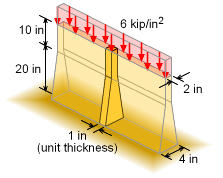Example Graphic A new tapered foundation is designed to support a distributed area load of 6 kip/in2. The top 10 inches has a constant width of 2 inches, but the bottom 20 inches tapers from 2 inches to 4 inches, as shown in the diagram. The foundation length is large when compared to the height or width. What is the vertical deflection of the foundation top? The foundation is constructed from concrete, E = 4×106 psi. Solution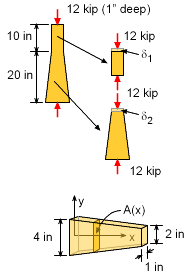Compression Load on Tapered Foundation The foundation can be analyzed using a 1 inch unit thickness which will have a load of      P = (6 kip/in2) (1 in) (2 in) = 12 kip This load will push down on the foundation from the top to the bottom. Next, it will be easier to analyze the deflection if the foundation is split into two sections, 1 and 2. The deflection of the top section can be determined by      δ1 = PL1/A1E1          = (-12,000) (10) / [(2)(4×106)] = -0.0150 in However, since the cross sectional area of the bottom section varies, the deflection needs to be integrated over its length using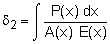The load P and stiffness E are constant, but the area, A changes over its length. The rate of change is linear, and can be modeled as      A(x) = 1 (4 - x/10) = (40 - x)/10 The variable of integration, x, is zero at the base where the area 4 in2 and goes to 20 in at the top of the bottom section where the area is 2 in2. This gives,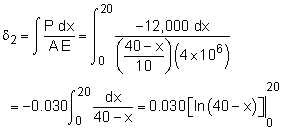= 0.030 (ln 20 - ln 40) = -0.02079 in The total deflection is the sum of both sections, δtotal = δ1 + δ2         = -0.01500 - 0.02079 = -0.03577 in Example 2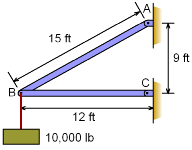Load Supported by Two Steel Rods Two steel rods, AB and BC, support a load, P of 10,000 lb. The diameter of rod AB and BC is 0.8 in and 1 in, respectively. Compute the magnitude of the angles through which rods AB and BC will rotate due to the given load. Assume Young's modulus for steel is 29×106 psi. Solution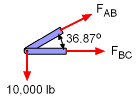Loads in Steel Rods From statics, the forces acting on members AB and BC can be determined as      ΣFy = 0      FAB sin36.87 - 10,000 = 0      FAB = 10,000 / sin36.87      FAB = 16,670 lb (Tension)      ΣFx = 0      FAB cos36.87 + FBC = 0      FBC = -16,670 cos36.87      FBC = -13,330 lb (Compression)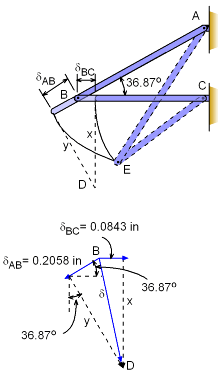Exaggerated View of Deformations and Rotations of Steel Rods Due to Applied Load The deformations of each rod due to FAB and  FBC are      δAB = FABLAB/ AAB E            = (16,670) (15) (12) / [π (0.8/2)2 (29×106)]            = 0.2058 in (lengthen)     δBC= FBCLBC/ ABC E            = (13,330) (12) (12) / [π (1/2)2 (29×106)]            = 0.0843 in (shorten) To analyze the effect of deformation on the movement of B it can be assumed that the members AB and BC are disconnected at B so that they undergo the deformations pictured (greatly exaggerated) in figure. To refasten, the members can be rotated about A and C to meet at E. However, the arc generated in these rotations are so small that they may be effectively replaced by straight lines drawn perpendicular to AB and BC respectively. These lines intersecting at D determine the effective final position of B. From the vector representation of the deformations δAB and δBC to a large scale it can be seen that the total movement of B is the vector BD or δ. From the vector diagram it is evident that the horizontal component of δ is      δh = δBC = 0.0843 in (right) However δh is also equal to algebraic sum of the horizontal components of δAB and the unknown length y, so that      δh = y sin36.87 - δAB cos36.87      0.0843 = y sin36.87 - 0.2058 cos36.87      y sin36.87 = 0.0843 + 0.1646      y = 0.2489 / sin36.87      y = 0.4148 in Now, x is the vertical component of δ, which is again the algebraic sum of the vertical components of δAB and y. So,      x = δv = δAB sin36.87 + y cos36.87      x = 0.2058 sin36.87 + 0.4148 cos36.87      x = 0.4553 in Now, the magnitude of angles through which members AB and BC rotate can be obtained as      αAB = y / LAB             = 0.4148 / [(15) (12)]             = 2.304×10-3 rad             = 0.1320 deg      αBC = x / LBC             = 0.4553 / [(12) (12)]             = 3.162×10-3 rad             = 0.1812 deg

Practice Homework and Test problems now available in the 'Eng Mechanics' mobile app
Includes over 400 problems with complete detailed solutions.
Available now at the Google Play Store and Apple App Store.